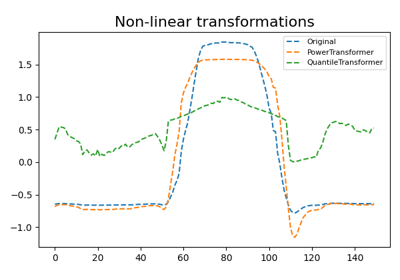# `pyts.preprocessing`.PowerTransformer¶

class `pyts.preprocessing.``PowerTransformer`(method='yeo-johnson', standardize=True)[source]

Apply a power transform sample-wise to make data more Gaussian-like.

Power transforms are a family of parametric, monotonic transformations that are applied to make data more Gaussian-like. This is useful for modeling issues related to heteroscedasticity (non-constant variance), or other situations where normality is desired.

Currently, PowerTransformer supports the Box-Cox transform and the Yeo-Johnson transform. The optimal parameter for stabilizing variance and minimizing skewness is estimated through maximum likelihood.

Box-Cox requires input data to be strictly positive, while Yeo-Johnson supports both positive or negative data.

By default, zero-mean, unit-variance normalization is applied to the transformed data.

Parameters: method : ‘yeo-johnson’ or ‘box-cox’ (default = ‘yeo-johnson’) The power transform method. Available methods are: ‘yeo-johnson’ , works with positive and negative values ‘box-cox’ , only works with strictly positive values standardize : boolean (default = True) Set to True to apply zero-mean, unit-variance normalization to the transformed output.

Notes

NaNs are treated as missing values: disregarded in `fit`, and maintained in `transform`.

References

  I.K. Yeo and R.A. Johnson, “A new family of power transformations to improve normality or symmetry.” Biometrika, 87(4), pp.954-959, (2000).
  G.E.P. Box and D.R. Cox, “An Analysis of Transformations”, Journal of the Royal Statistical Society B, 26, 211-252 (1964).

Examples

```>>> import numpy as np
>>> from pyts.preprocessing import PowerTransformer
>>> X = [[1, 3, 4], [2, 2, 5]]
>>> pt = PowerTransformer()
>>> print(pt.transform(X))
[[-1.316...  0.209...  1.106...]
[-0.707... -0.707...  1.414...]]
```

Methods

 `__init__`([method, standardize]) Initialize self. `fit`([X, y]) Pass. `fit_transform`(X[, y]) Fit to data, then transform it. `get_params`([deep]) Get parameters for this estimator. `set_params`(**params) Set the parameters of this estimator. `transform`(X) Transform the data.
`__init__`(method='yeo-johnson', standardize=True)[source]

Initialize self. See help(type(self)) for accurate signature.

`fit`(X=None, y=None)[source]

Pass.

Parameters: X Ignored y Ignored self : object
`fit_transform`(X, y=None, **fit_params)

Fit to data, then transform it.

Fits transformer to X and y with optional parameters fit_params and returns a transformed version of X.

Parameters: X : array-like, shape = (n_samples, n_timestamps) Univariate time series. y : None or array-like, shape = (n_samples,) (default = None) Target values (None for unsupervised transformations). **fit_params : dict Additional fit parameters. X_new : array Transformed array.
`get_params`(deep=True)

Get parameters for this estimator.

Parameters: deep : bool, default=True If True, will return the parameters for this estimator and contained subobjects that are estimators. params : dict Parameter names mapped to their values.
`set_params`(**params)

Set the parameters of this estimator.

The method works on simple estimators as well as on nested objects (such as `Pipeline`). The latter have parameters of the form `<component>__<parameter>` so that it’s possible to update each component of a nested object.

Parameters: **params : dict Estimator parameters. self : estimator instance Estimator instance.
`transform`(X)[source]

Transform the data.

Parameters: X : array-like, shape = (n_samples, n_timestamps) Data to transform. X_new : array-like, shape = (n_samples, n_timestamps) Transformed data.

## Examples using `pyts.preprocessing.PowerTransformer`¶Transformers